### Power System analysis

Graph Theory:

• Graph Theory is used to predict the behaviour of networks for analysis.

• For small networks, Node and Mesh analysis is more convenient.

• If the network is more complex then analysis of currents and voltages is quite difficult with the traditional Nodal and Mesh Analysis.

Let us discuss some terminology used in the Graph Theory concepts:

1. Element: Each line segment or an arc in a network graph is called an Element.
In order to analyze a network, Voltage sources are replaced as short circuit and Current sources are replaced as Open circuit.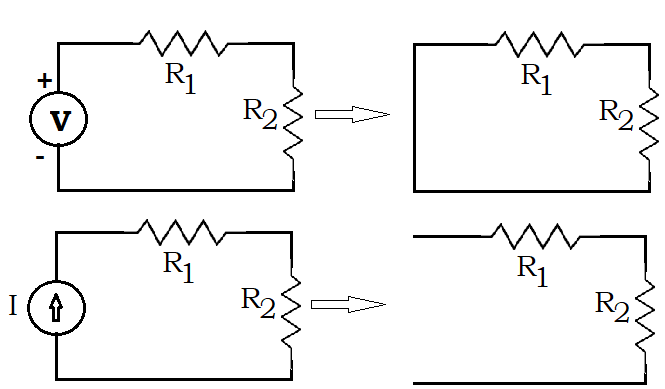1. Node or Vertex: The terminal of an element is called as Node or vertex.

2. Edge: An element of a graph is called as Edge.

3. Degree: Number of edges connected to a node is called as Degree of a graph.

4. Rank: The rank of a graph is given as n-1, where n is number of nodes.

5. Sub Graph: Subsets of elements of a graph is called as Syb graph.

6. Planar Graph: If there are no cross over of edges in a graph, then the graph is called Planar graph.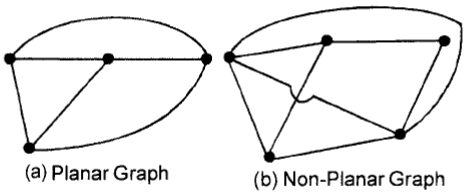1. Non Planar Graph: If there are cross over of edges in a graph, then the graph is called Non Planar graph.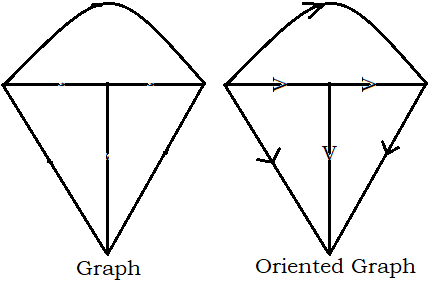1. Closed path or Loop: If the edge starts from one node and return to the same node we call it as Closed path or Loop.1. Oriented Graph: If in a graph the directions of currents are marked on each element, then we call the graph as Oriented Graph.

2. Tree: A tree is nothing but a graph with all the nodes present but doesn’t have any loops.

Number possible trees can be drawn for a Graph.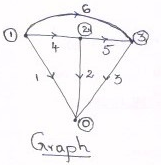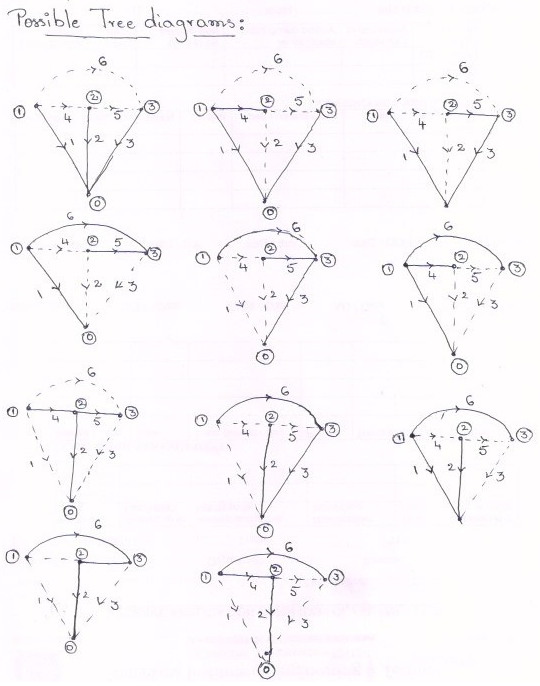1. Twigs: The branches of a tree are called Twigs.

2. Links or Chords: The remaining branches of a tree are called Links or Chords.

3. Co-Tree: The compliment of tree is Co-Tree.

Number of branches, b = n - 1,

Where b is No: of branches, n is No: of nodes

Number of Links, l = e - b,

Where l is No: of links, e is No: of elements.

1. Basic Loop or Independent Loop: In a graph, if loop contains only one link, then it is called as a Basic loop or independent loop.